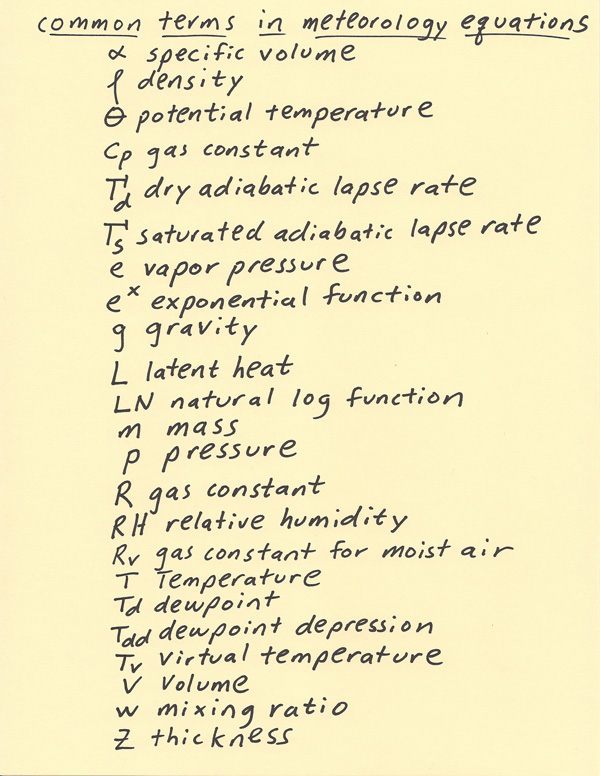[--MAIN HOME--] [--ALL HABYHINTS--] [--FACEBOOK PAGE--]

 HABYTIME MINI LECTURE 60: METR. EQUATION TERMS

METEOROLOGIST JEFF HABY

Below is a list of common terms that will be found in meteorology readings, especially when the solving of meteorology equations is involved. A basic definition of each is given. At the bottom of this writing is how the symbols look.

Specific volume: volume divided by mass. It is the inverse of density since density is mass divided by volume

Density: mass divided by volume. Example units density is given in are kg/m^3 and g/cm^3

Potential Temperature: Temperature of air when brought to the 1000 mb pressure surface. Common units are in Kelvins. Kelvins is Celsius temperature + 273.15

Gas Constants: There are several different gas constants such as at constant pressure Cp and constant volume Cv. This constant often comes up when solving thermodynamic equations. There is also the gas constant R.

Dry Adiabatic Lapse Rate: This is the rate of cooling of a rising unsaturated parcel of air. This rate has the same number as gravity but with units of degrees C / kilometer. The dry adiabatic lapse rate is a cooling of 9.8 C/km. When air is sinking it is the same rate except the temperature warms 9.8 C/km.

Saturated (Wet) Adiabatic Lapse Rate: This is the rate of cooling of a rising saturated parcel of air. Some of the adiabatic cooling is offset by condensational warming as moisture is condensed out of the air. The saturated lapse rate is not a constant since the amount of condensational warming is a function of temperature. The saturated adiabatic lapse rate varies from about 4 to close to 9.8 C/km. It is closer to 4 C/km in very warm saturated air and closer to 9.8 C/km in very cold saturated air.

Vapor Pressure (e): This is the contribution to the air pressure from water vapor alone. The vapor pressure (e) is expressed in millibars. The saturation vapor pressure (es) is the vapor pressure produced from saturated air.

e^x: Not to be confused with vapor pressure. This is the exponential math operation.

Gravity (g): 9.8 m/s^2. Gravity is a force produced by attractions of mass to one another.

Latent Heat (L): This is the warming (release) or cooling (absorption) of heat by processes such as melting, freezing, evaporation, condensation, deposition, sublimation

LN: This is the natural log math operation

Mass (m): Amount of material that makes up something. It is commonly expressed in grams or kilograms.

Pressure (P): This is the force per unit area. In meteorology it is usually in reference to air pressure.

Gas Constant (R): This term commonly comes up in thermodynamic equations. There are different versions of the gas constant depending on the situation being analyzed.

RH: This is the relative humidity. Two formulas for finding the RH are: RH = (e/es)*100% and (w/ws)*100%

Rv: Gas constant for moist air = 461 J/(kg*K)

T: Temperature

Td: Dewpoint. This is temperature the air needs to be cooled to in order for the air to reach saturation (RH=100%)

Tdd: Dewpoint depression. This is the Temperature minus the Dewpoint

Tv: Virtual temperature. Virtual temperature is the temperature that perfectly dry air would need to have to have the same density as the air with moisture. Dry air needs to be warmed in order to have the same density as moist air at the same temperature since dry air is denser than moist air at the same temperature. Virtual temperature is used to simplify meteorology equations.

Volume (V): Cubic measurement of a substance

Mixing ratio (w): This is the grams of moisture in the air divided by the total mass of the air in kilograms. The saturation mixing ratio (ws) is the mixing ratio of saturated air.

Thickness (Z): This is the vertical distance between two pressure levels.